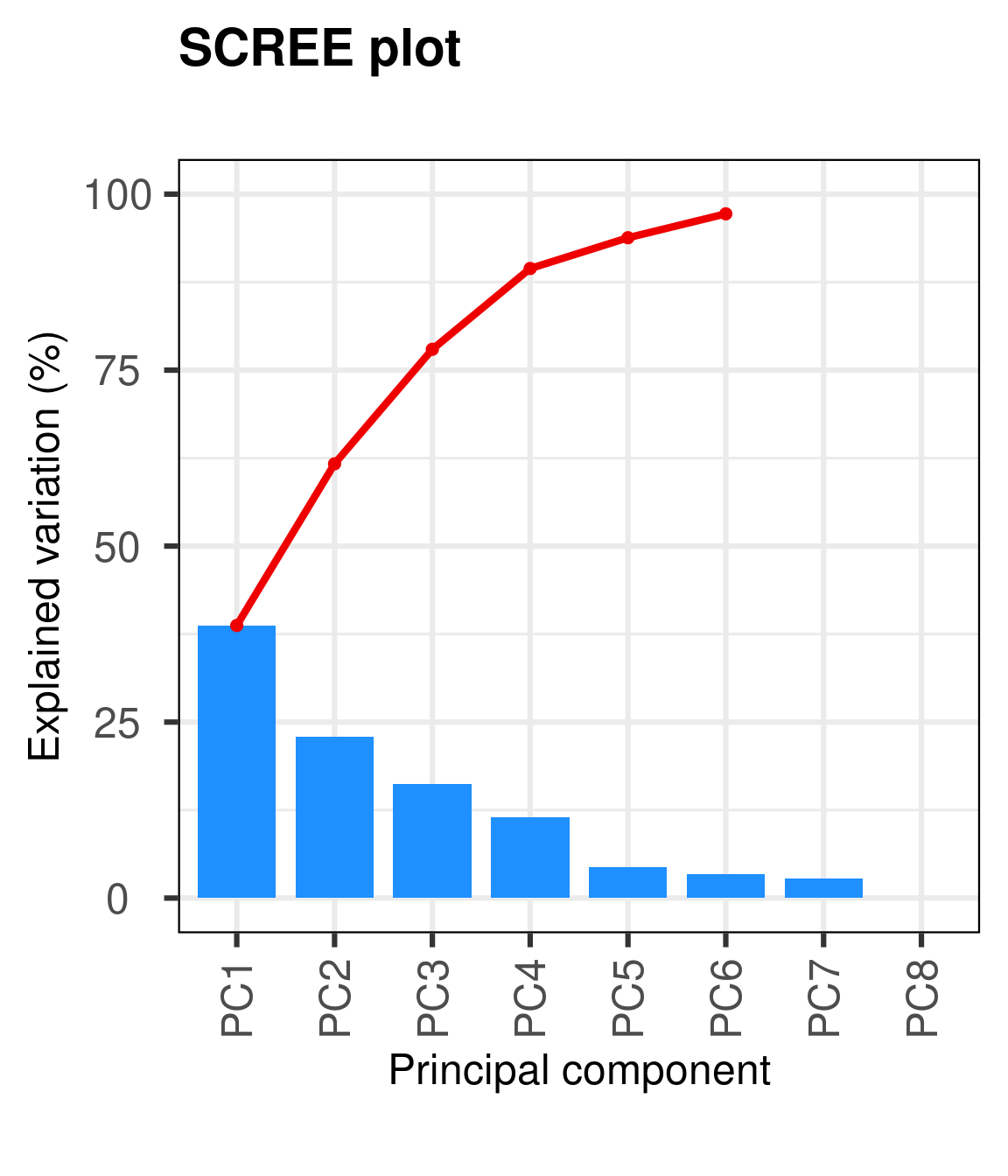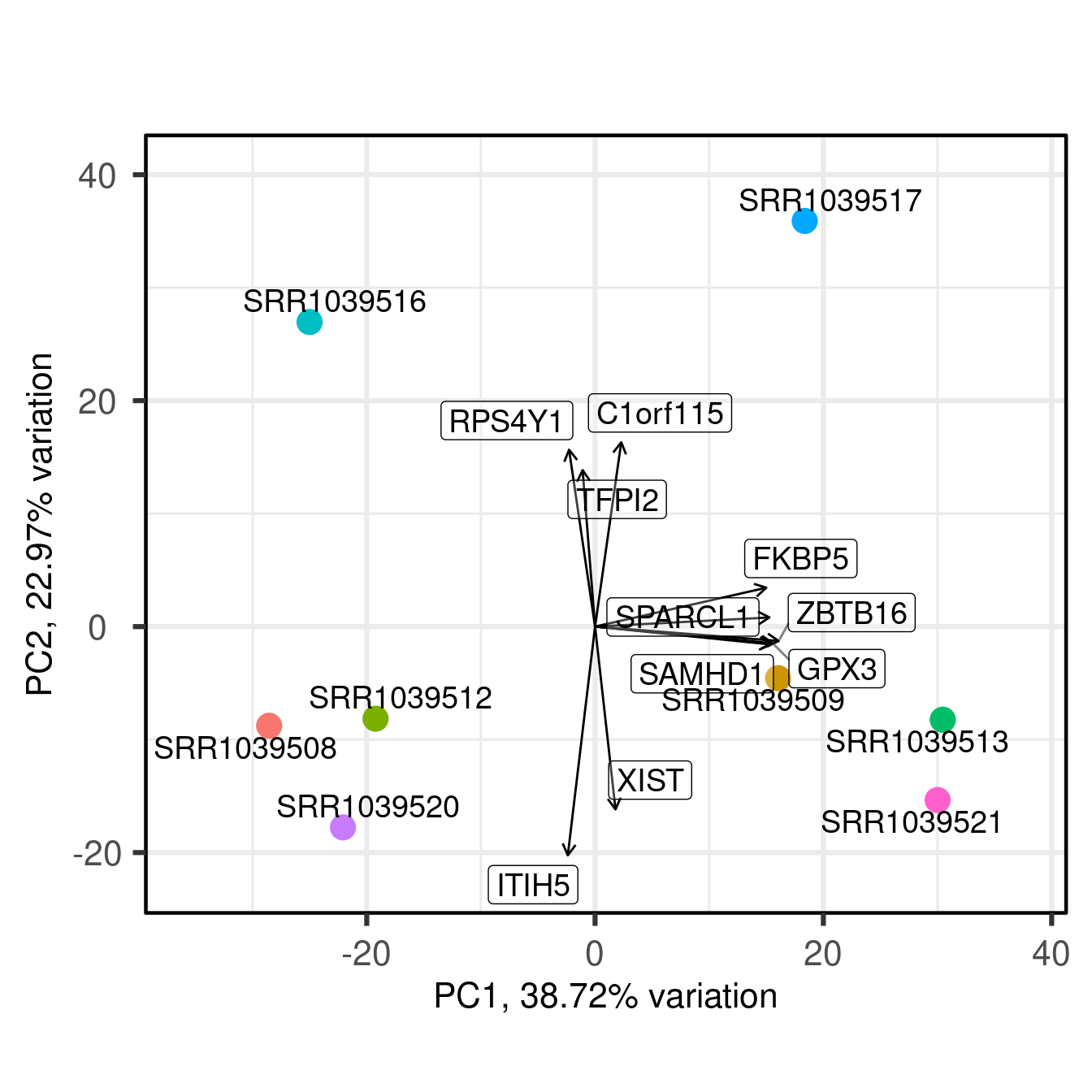# 1 Introduction

Principal Component Analysis (PCA) is a very powerful technique that has wide applicability in data science, bioinformatics, and further afield. It was initially developed to analyse large volumes of data in order to tease out the differences/relationships between the logical entities being analysed. It extracts the fundamental structure of the data without the need to build any model to represent it. This ‘summary’ of the data is arrived at through a process of reduction that can transform the large number of variables into a lesser number that are uncorrelated (i.e. the ‘principal components’), while at the same time being capable of easy interpretation on the original data (Blighe and Lun 2019) (Blighe 2013).

PCAtools provides functions for data exploration via PCA, and allows the user to generate publication-ready figures. PCA is performed via BiocSingular (Lun 2019) - users can also identify optimal number of principal components via different metrics, such as elbow method and Horn’s parallel analysis (Horn 1965) (Buja and Eyuboglu 1992), which has relevance for data reduction in single-cell RNA-seq (scRNA-seq) and high dimensional mass cytometry data.

# 2 Installation

``````    if (!requireNamespace('BiocManager', quietly = TRUE))
install.packages('BiocManager')

BiocManager::install('PCAtools')``````

Note: to install development version direct from GitHub:

``````    if (!requireNamespace('devtools', quietly = TRUE))
install.packages('devtools')

devtools::install_github('kevinblighe/PCAtools')``````

## 2.2 2. Load the package into R session

``    library(PCAtools)``

# 3 Quick start: DESeq2

For this example, we will follow the tutorial (from Section 3.1) of RNA-seq workflow: gene-level exploratory analysis and differential expression. Specifically, we will load the ‘airway’ data, where different airway smooth muscle cells were treated with dexamethasone.

``````  library(airway)
library(magrittr)

data('airway')
airway\$dex %<>% relevel('untrt')``````

Annotate the Ensembl gene IDs to gene symbols:

``````  ens <- rownames(airway)

library(org.Hs.eg.db)
symbols <- mapIds(org.Hs.eg.db, keys = ens,
column = c('SYMBOL'), keytype = 'ENSEMBL')
symbols <- symbols[!is.na(symbols)]
symbols <- symbols[match(rownames(airway), names(symbols))]
rownames(airway) <- symbols
keep <- !is.na(rownames(airway))
airway <- airway[keep,]``````

Normalise the data and transform the normalised counts to variance-stabilised expression levels:

``````  library('DESeq2')

dds <- DESeqDataSet(airway, design = ~ cell + dex)
dds <- DESeq(dds)
vst <- assay(vst(dds))``````

## 3.1 Conduct principal component analysis (PCA):

``  p <- pca(vst, metadata = colData(airway), removeVar = 0.1)``
``## -- removing the lower 10% of variables based on variance``

## 3.2 A scree plot

``  screeplot(p, axisLabSize = 18, titleLabSize = 22)``Figure 1: A scree plot

## 3.3 A bi-plot

Different interpretations of the biplot exist. In the OMICs era, for most general users, a biplot is a simple representation of samples in a 2-dimensional space, usually focusing on just the first two PCs:

``  biplot(p)``

However, the original definition of a biplot by Gabriel KR (Gabriel 1971) is a plot that plots both variables and observations (samples) in the same space. The variables are indicated by arrows drawn from the origin, which indicate their ‘weight’ in different directions. We touch on this later via the plotLoadings function.

``````  biplot(p, showLoadings = TRUE,Figure 2: A bi-plot

# 4 Quick start: Gene Expression Omnibus (GEO)

Here, we will instead start with data from Gene Expression Omnibus. We will load breast cancer gene expression data with recurrence free survival (RFS) from Gene Expression Profiling in Breast Cancer: Understanding the Molecular Basis of Histologic Grade To Improve Prognosis.

First, let’s read in and prepare the data:

``````  library(Biobase)
library(GEOquery)

# load series and platform data from GEO
gset <- getGEO('GSE2990', GSEMatrix = TRUE, getGPL = FALSE)
mat <- exprs(gset[])

# remove Affymetrix control probes
mat <- mat[-grep('^AFFX', rownames(mat)),]

# extract information of interest from the phenotype data (pdata)
idx <- which(colnames(pData(gset[])) %in%
c('relation', 'age:ch1', 'distant rfs:ch1', 'er:ch1',
'time rfs:ch1'))
row.names = rownames(pData(gset[])))

# tidy column names
'Size', 'Time.RFS')

# prepare certain phenotypes of interest
levels = c(0,1))
levels = c(0,1))
levels = c('ER-', 'ER+'))
levels = c(1,2,3))

# remove samples from the pdata that have any NA value

# filter the expression data to match the samples in our pdata

# check that sample names match exactly between pdata and expression data
``##  TRUE``
``  p <- pca(mat, metadata = metadata, removeVar = 0.1)``
``## -- removing the lower 10% of variables based on variance``
``  biplot(p)``
``  biplot(p, showLoadings = TRUE, lab = NULL)``